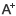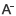# 苏教版六年级上册数学训练与检测答案(第111页-116页)日期：2020-12-10     来源：www.vqunkong.com    作者：智学网    浏览：313    评论：0

1：⑴涂色涂8格；画线画1格。

⑵＜　　＞　　＞

⑶13∶12　　13/25　　125

⑷16　　25

⑸9　　25米　　6

2：⑴C　　⑵A　　⑶B

3：3/8÷5/16=6/5　　9/10÷3/5=3/2　　8/9÷20/27=6/5

1：⑴A　　⑵A　　⑶B

2：⑴7　　42　　28

⑵5/4

⑶31

3：1÷3/8=8/3　　1/3+1/4=7/12　　2-2/5=8/5　　4/5*1/5=4/25

4：（1）（3/4－2/3）÷1/5

=（9/12-8/12）×5

=1/12×5

=5/12

（2）（3/8+2/17）×8+1/17

=3/8×8+2/17×8+1/17

=3+

=3+1

=4

（3） 4/5×7/8+7/8÷5 　　 （4） ÷3/5
= 4/5×7/8+7/8×1/5 　　　　 = ÷3/5
= 7/8× 　　　　　　 = 3/5÷3/5
= 7/8×1 　　　　　　　　　　 = 1
= 7/8

（5） 1－5/8÷25/28－3/10 　　 （6） [5/6－]÷4/9
= 1－7/10－3/10 　　　　　　 = [5/6－1/6]÷4/9
= 1－ 　　 　　　　 = 2/3×9/4
= 1－1 　　 　　　　　　　　 = 3/2
= 0
5:9×1/4+1/2

=9/4+1/2

=11/4（米）

答：两次一共用去11/4米。

6：70×（1-13/35）

=70×22/35

=44（枚）

答：银牌和铜牌一共有44枚。

7：1800×1/3－1800×2/9

=600－400

=200（吨）

答：第二天比第一天多运走200吨。

8:

（2600－200）÷（1+5）

=2400÷6

=400（毫升）

400+200=600（毫升）

答：每一个小瓶装药水400毫升，每一个大瓶装药水600毫升。

1：⑴C　　⑵B　　⑶B　　⑷C

2：⑴100　　50

⑵16　　30　　80

⑶3.5

⑷110

⑸72　　450

⑹0.166＜1/6＜16.7%＜1.6

3：

（1）x+30%x=52

1.3x=52

x=40

（2）2/3x+3/4x=1/6

17/12x=1/6

x=2/17

4.1.8÷（22.2+1.8）×100%=7.5%

5.3000×1%=30（元）

6.255÷3÷85%=100（元）

7.30÷（1/2-20%）=100（千克）

8.解：设松树栽了x棵，柏树栽了25%x棵。

x+25%x=120

x=96

25%x=25%×96=24（棵）

答：松树栽了96棵，柏树栽了24棵。

0相关评论